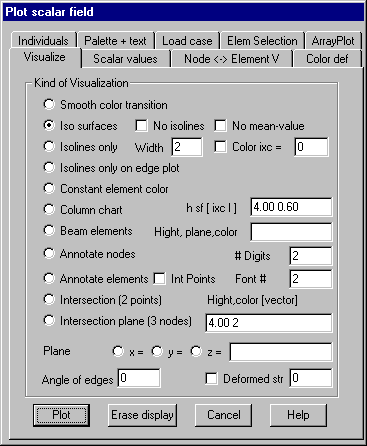Visualize: Specify the kind of visualization for scalar fields

On this property page you can specify the kind of visualization to be used for subsequent plots. After setting the appropriate option button „Plot“ starts the graphics. Button „Erase display“ can be used to erase all display lists.

Following dialog shows the available options:Smooth color transition

Color values are assigned to the nodes of the elements corresponding to the scalar values belonging to the nodes. Among these points a continuous color transition is used. The assignment of color indices to scalar data is done within property page Scalar values.

Iso surfaces

At first, isolines are calculated for individual scalar values. Next the area between to adjacent isolines is filled with a constant color. Optionally isolines can also be plotted. The calculation of isolines is done by linear interpolation using the scalar values calculated for the nodes of the triangles that approximate the elements. If nodes belong to more than one element, the median of the values resulting from the different elements is calculated. If option “No median” is set, no median values are calculated, so there is no continuously transition on element edges.

Iso lines only

No color filling between iso lines is done. Iso lines are plotted using a color corresponding to the scalar value of the iso line. If option “Color ixc” is checked, all iso lines are plotted with the given color in the input field. In the input field for width the width for plotting the iso lines may be given. With this option a hidden line plot is done.

Iso lines only on edge plot

The difference of this option to the option before is, that no hidden line plot is done; only iso lines and element edges are plotted.

Constant element color

With this option set all element surfaces are filled with a constant color. The color is determined by the associated scalar value of the element.

Column chart

Perpendicular to the element surfaces columns are plotted with heights proportional to the elements scalar value. In the input field height h for the largest column, scaling factor sf for the columns and optionally a constant color index ixc and a flag l (shading flag) must be given. The scaling factor determines the ratio between the width of the columns and the edge length of the elements. If there is no color given the color is determined by the scalar value of the element. For a flag l > 0 the columns are shaded.

Beam elements

This option is intended for bar and beam elements with scalar fields of type ktyp = 30 – 33. Perpendicular to the axis of the element the distribution of the scalar values is plotted as a colored bar in the given plane. In the input field, following parameters must be given: Plotted height for the maximal scalar value, 3 components of a vector lying on the plane in which the distribution should be plotted and the color to be used for the plot. The vector must not be parallel to a beam axis. Several plots are superimposed.

Annotate nodes

With this option all nodes contained within the current selection are annotated with associated scalar values. The number of digits following the decimal point and the font index (1-3) for text height and color must be given.

Annotate elements

This option shows the associated scalar value for the elements at the center of gravity. Further options are the same as with option “Annotate nodes”. If scalar values are given for integration points, option “Integr point” can be checked; in this case the integration points are annotated with corresponding scalar values.

Intersection (2 points)

With this option 2 points defining the end points of an intersection line must be graphically selected within the current view after pressing button „Plot“. On each intersection line all scalar values at the intersection of the line with element edges are calculated using linear interpolation. The scalar values are plotted along the intersection line. Within the input field maximum height for scalar distribution, color index for filling and optionally 3 components of a direction vector for the surface can be given. In case there is no direction vector given the plot is done perpendicular to the elements surface. Several intersection lines can be selected sequentially until right mouse button is pressed.

Intersection plane (3 points)

By selecting 3 points after pressing button “Plot”, a spatial intersection plane is generated. Scalar values are calculated at all intersections between this plane and element edges. Scalar values are plotted within this intersection plane. Additional parameters to be specified are the same as with option “Intersection”.

Plane x, y, z

This options do intersections parallel to global coordinate system planes. Within the input field several values for x,y or z-coordinates of these intersection planes can be given.

Angle of edges

If an angle > 0 (degrees) is given only these element edges are plotted whose angles between the normal vectors of adjacent element surfaces are greater than this value.

Deformed structure

With this option set the plot is done within the deformed element structure. This is only applicable if there is also a vector field with node displacements loaded and activated by the command Vector field. The maximum length for displaying a displacement must be given.